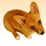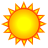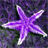# \$\$NCOLS + \$\$ROWMAP

5695
5
01-20-2012 05:44 PMby
New Contributor
How do we implement \$\$NCOLS + \$\$ROWMAP in map calculator? I would appreciate any advice. My email: yong.q.tian@gmail.com
Tags (4)
1 Solution

Accepted SolutionsbyMVP Esteemed Contributor

Here's an implementation of   William Huber's neat idea of using the FlowAccumulation tool to generate xmap and  ymap. This uses the current processing environment, it will generate an error if the arcpy.env.extent or cellSize are not explicitly set.

(this code has a bug fixed - thanks Cameron!

`from arcpy.sa import *from arcpy import env as E# Calculate \$\$NROWS and \$\$NCOLS from current environmentcellSize = float(E.cellSize)nrows = int((E.extent.YMax - E.extent.YMin) / float(E.cellSize))ncols = int((E.extent.XMax - E.extent.XMin)  / float(E.cellSize))# Bill Huber's method for \$\$XMAP and \$\$YMAP: "1" flows "right", "64" (63+1) flows "up"tmpg = CreateConstantRaster(1)xmap = (FlowAccumulation(tmpg) + 0.5) * cellSize + E.extent.XMinymap = (FlowAccumulation(tmpg + 63) + 0.5) * cellSize + E.extent.YMin# applying the same method for \$\$ROWMAP and \$\$COLMAPcolmap = Int(FlowAccumulation(tmpg))rowmap = Int(FlowAccumulation(tmpg + 3))  # flowdir "4" is "down" (top row is 0)`
5 RepliesRegular Contributor III
Unsuported in new v10.0 Map Algebra! Although you can get \$\$NCOLS through the describe property in Python or the GetRasterProperties toolbox tool.

You can still use the good ole' Map Algebra sytax in v10 though:

`arcpy.gp.SingleOutputMapAlgebra_sa(blah, blah)`New Contributor
Thanks for this! I'm new to both rasters and Python so code like this is a HUGE help. However, I think there's a small bug.

Shouldn't the xmap setting be adding XMin instead of YMin at the end? I.e.,
xmap = (FlowAccumulation(tmpg) + 0.5) * cellSize + E.extent.XMin

Thanks again for the code, very useful.byMVP Esteemed Contributor

Here's an implementation of   William Huber's neat idea of using the FlowAccumulation tool to generate xmap and  ymap. This uses the current processing environment, it will generate an error if the arcpy.env.extent or cellSize are not explicitly set.

(this code has a bug fixed - thanks Cameron!

`from arcpy.sa import *from arcpy import env as E# Calculate \$\$NROWS and \$\$NCOLS from current environmentcellSize = float(E.cellSize)nrows = int((E.extent.YMax - E.extent.YMin) / float(E.cellSize))ncols = int((E.extent.XMax - E.extent.XMin)  / float(E.cellSize))# Bill Huber's method for \$\$XMAP and \$\$YMAP: "1" flows "right", "64" (63+1) flows "up"tmpg = CreateConstantRaster(1)xmap = (FlowAccumulation(tmpg) + 0.5) * cellSize + E.extent.XMinymap = (FlowAccumulation(tmpg + 63) + 0.5) * cellSize + E.extent.YMin# applying the same method for \$\$ROWMAP and \$\$COLMAPcolmap = Int(FlowAccumulation(tmpg))rowmap = Int(FlowAccumulation(tmpg + 3))  # flowdir "4" is "down" (top row is 0)`byEsri Esteemed Contributor

It's always cool to see some of William Huber‌ ideas revive!

Thanx for sharing Curtis PricebyMVP Esteemed Contributor

No problem, I was just resurrecting some old posts of mine, getting them tagged and placed in GeoNet where they will be easier to find.